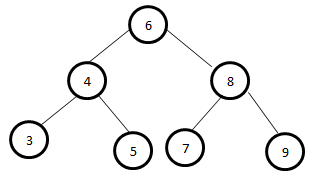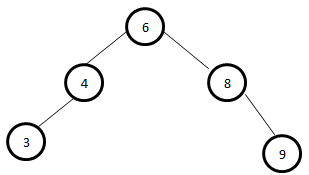# Sum of all the levels in a Binary Search Tree

Given a Binary Search Tree, the task is to find the horizontal sum of the nodes that are in the same level.

Examples:

Input:Output:
6
12
24

Input:Output:
6
12
12

## Recommended: Please try your approach on {IDE} first, before moving on to the solution.

Approach: Find the height of the given binary tree then the number of levels in the tree will be levels = height + 1. Now create an array sum[] of size levels where sum[i] will store the sum of all the nodes at the ith level. In order to update this array, write a recursive function that add the current node’s data at sum[level] where level is the level of the current node and then recursively call the same method for the child nodes with level as level + 1.

Below is the implementation of the above approach:

## C++

 `// C++ implementation of the approach ` `#include ` `#include ` `using` `namespace` `std; ` ` `  `// A Binary Tree Node ` `struct` `Node { ` `    ``int` `data; ` `    ``struct` `Node *left, *right; ` `}; ` ` `  `// Utility function to create a new tree node ` `Node* newNode(``int` `data) ` `{ ` `    ``Node* temp = ``new` `Node; ` `    ``temp->data = data; ` `    ``temp->left = temp->right = NULL; ` `    ``return` `temp; ` `} ` ` `  `// Utility function to print ` `// the contenets of an array ` `void` `printArr(``int` `arr[], ``int` `n) ` `{ ` `    ``for` `(``int` `i = 0; i < n; i++) ` `        ``cout << arr[i] << endl; ` `} ` ` `  `// Function to return the height ` `// of the binary tree ` `int` `getHeight(Node* root) ` `{ ` `    ``if` `(root->left == NULL && root->right == NULL) ` `        ``return` `0; ` ` `  `    ``int` `left = 0; ` `    ``if` `(root->left != NULL) ` `        ``left = getHeight(root->left); ` ` `  `    ``int` `right = 0; ` `    ``if` `(root->right != NULL) ` `        ``right = getHeight(root->right); ` ` `  `    ``return` `(max(left, right) + 1); ` `} ` ` `  `// Recursive function to update sum[] array ` `// such that sum[i] stores the sum ` `// of all the elements at ith level ` `void` `calculateLevelSum(Node* node, ``int` `level, ``int` `sum[]) ` `{ ` `    ``if` `(node == NULL) ` `        ``return``; ` ` `  `    ``// Add current node data to the sum ` `    ``// of the current node's level ` `    ``sum[level] += node->data; ` ` `  `    ``// Recursive call for left and right sub-tree ` `    ``calculateLevelSum(node->left, level + 1, sum); ` `    ``calculateLevelSum(node->right, level + 1, sum); ` `} ` ` `  `// Driver code ` `int` `main() ` `{ ` `    ``// Create the binary tree ` `    ``Node* root = newNode(6); ` `    ``root->left = newNode(4); ` `    ``root->right = newNode(8); ` `    ``root->left->left = newNode(3); ` `    ``root->left->right = newNode(5); ` `    ``root->right->left = newNode(7); ` `    ``root->right->right = newNode(9); ` ` `  `    ``// Count of levels in the ` `    ``// given binary tree ` `    ``int` `levels = getHeight(root) + 1; ` ` `  `    ``// To store the sum at every level ` `    ``int` `sum[levels] = { 0 }; ` `    ``calculateLevelSum(root, 0, sum); ` ` `  `    ``// Print the required sums ` `    ``printArr(sum, levels); ` ` `  `    ``return` `0; ` `} `

## Java

 `// Java implementation of the approach  ` `class` `Sol ` `{ ` `     `  `// A Binary Tree Node  ` `static` `class` `Node  ` `{  ` `    ``int` `data;  ` `    ``Node left, right;  ` `};  ` ` `  `// Utility function to create a new tree node  ` `static` `Node newNode(``int` `data)  ` `{  ` `    ``Node temp = ``new` `Node();  ` `    ``temp.data = data;  ` `    ``temp.left = temp.right = ``null``;  ` `    ``return` `temp;  ` `}  ` ` `  `// Utility function to print  ` `// the contenets of an array  ` `static` `void` `printArr(``int` `arr[], ``int` `n)  ` `{  ` `    ``for` `(``int` `i = ``0``; i < n; i++)  ` `        ``System.out.print(arr[i]+ ``" "` `);  ` `}  ` ` `  `// Function to return the height  ` `// of the binary tree  ` `static` `int` `getHeight(Node root)  ` `{  ` `    ``if` `(root.left == ``null` `&& root.right == ``null``)  ` `        ``return` `0``;  ` ` `  `    ``int` `left = ``0``;  ` `    ``if` `(root.left != ``null``)  ` `        ``left = getHeight(root.left);  ` ` `  `    ``int` `right = ``0``;  ` `    ``if` `(root.right != ``null``)  ` `        ``right = getHeight(root.right);  ` ` `  `    ``return` `(Math.max(left, right) + ``1``);  ` `}  ` ` `  `// Recursive function to update sum[] array  ` `// such that sum[i] stores the sum  ` `// of all the elements at ith level  ` `static` `void` `calculateLevelSum(Node node, ``int` `level, ``int` `sum[])  ` `{  ` `    ``if` `(node == ``null``)  ` `        ``return``;  ` ` `  `    ``// Add current node data to the sum  ` `    ``// of the current node's level  ` `    ``sum[level] += node.data;  ` ` `  `    ``// Recursive call for left and right sub-tree  ` `    ``calculateLevelSum(node.left, level + ``1``, sum);  ` `    ``calculateLevelSum(node.right, level + ``1``, sum);  ` `}  ` ` `  `// Driver code  ` `public` `static` `void` `main(String args[]) ` `{  ` `    ``// Create the binary tree  ` `    ``Node root = newNode(``6``);  ` `    ``root.left = newNode(``4``);  ` `    ``root.right = newNode(``8``);  ` `    ``root.left.left = newNode(``3``);  ` `    ``root.left.right = newNode(``5``);  ` `    ``root.right.left = newNode(``7``);  ` `    ``root.right.right = newNode(``9``);  ` ` `  `    ``// Count of levels in the  ` `    ``// given binary tree  ` `    ``int` `levels = getHeight(root) + ``1``;  ` ` `  `    ``// To store the sum at every level  ` `    ``int` `sum[]=``new` `int``[levels];  ` `    ``calculateLevelSum(root, ``0``, sum);  ` ` `  `    ``// Print the required sums  ` `    ``printArr(sum, levels);  ` `}  ` `}  ` ` `  `// This code is contributed by andrew1234 `

## Python

 `# Python implementation of above algorithm ` ` `  `# Utility class to create a node  ` `class` `Node:  ` `    ``def` `__init__(``self``, key):  ` `        ``self``.data ``=` `key  ` `        ``self``.left ``=` `self``.right ``=` `None` `         `  `# Utility function to create a tree node ` `def` `newNode( data): ` ` `  `    ``temp ``=` `Node(``0``) ` `    ``temp.data ``=` `data ` `    ``temp.left ``=` `temp.right ``=` `None` `    ``return` `temp ` ` `  `# Utility function to print ` `# the contenets of an array ` `def` `printArr(arr, n): ` ` `  `    ``i ``=` `0` `    ``while` `( i < n): ` `        ``print``( arr[i]) ` `        ``i ``=` `i ``+` `1` ` `  `# Function to return the height ` `# of the binary tree ` `def` `getHeight(root): ` ` `  `    ``if` `(root.left ``=``=` `None` `and` `root.right ``=``=` `None``): ` `        ``return` `0` ` `  `    ``left ``=` `0` `    ``if` `(root.left !``=` `None``): ` `        ``left ``=` `getHeight(root.left) ` ` `  `    ``right ``=` `0` `    ``if` `(root.right !``=` `None``): ` `        ``right ``=` `getHeight(root.right) ` ` `  `    ``return` `(``max``(left, right) ``+` `1``) ` ` `  `sum` `=` `[] ` ` `  `# Recursive function to update sum[] array ` `# such that sum[i] stores the sum ` `# of all the elements at ith level ` `def` `calculateLevelSum(node, level): ` `     `  `    ``global` `sum` `    ``if` `(node ``=``=` `None``): ` `        ``return` ` `  `    ``# Add current node data to the sum ` `    ``# of the current node's level ` `    ``sum``[level] ``+``=` `node.data ` ` `  `    ``# Recursive call for left and right sub-tree ` `    ``calculateLevelSum(node.left, level ``+` `1``) ` `    ``calculateLevelSum(node.right, level ``+` `1``) ` ` `  ` `  `# Driver code ` ` `  `# Create the binary tree ` `root ``=` `newNode(``6``) ` `root.left ``=` `newNode(``4``) ` `root.right ``=` `newNode(``8``) ` `root.left.left ``=` `newNode(``3``) ` `root.left.right ``=` `newNode(``5``) ` `root.right.left ``=` `newNode(``7``) ` `root.right.right ``=` `newNode(``9``) ` ` `  `# Count of levels in the ` `# given binary tree ` `levels ``=` `getHeight(root) ``+` `1` ` `  `# To store the sum at every level ` `sum` `=` `[``0``] ``*` `levels  ` `calculateLevelSum(root, ``0``) ` ` `  `# Print the required sums ` `printArr(``sum``, levels) ` ` `  `# This code is contributed by Arnab Kundu `

## C#

 `// C# implementation of the approach  ` `using` `System;  ` `class` `GFG  ` `{  ` `     `  `// A Binary Tree Node  ` `public` `class` `Node  ` `{  ` `    ``public` `int` `data;  ` `    ``public` `Node left, right;  ` `};  ` ` `  `// Utility function to create a new tree node  ` `static` `Node newNode(``int` `data)  ` `{  ` `    ``Node temp = ``new` `Node();  ` `    ``temp.data = data;  ` `    ``temp.left = temp.right = ``null``;  ` `    ``return` `temp;  ` `}  ` ` `  `// Utility function to print  ` `// the contenets of an array  ` `static` `void` `printArr(``int` `[]arr, ``int` `n)  ` `{  ` `    ``for` `(``int` `i = 0; i < n; i++)  ` `        ``Console.WriteLine(arr[i]);  ` `}  ` ` `  `// Function to return the height  ` `// of the binary tree  ` `static` `int` `getHeight(Node root)  ` `{  ` `    ``if` `(root.left == ``null` `&&  ` `        ``root.right == ``null``)  ` `        ``return` `0;  ` ` `  `    ``int` `left = 0;  ` `    ``if` `(root.left != ``null``)  ` `        ``left = getHeight(root.left);  ` ` `  `    ``int` `right = 0;  ` `    ``if` `(root.right != ``null``)  ` `        ``right = getHeight(root.right);  ` ` `  `    ``return` `(Math.Max(left, right) + 1);  ` `}  ` ` `  `// Recursive function to update sum[] array  ` `// such that sum[i] stores the sum  ` `// of all the elements at ith level  ` `static` `void` `calculateLevelSum(Node node, ``int` `level,  ` `                                         ``int` `[]sum)  ` `{  ` `    ``if` `(node == ``null``)  ` `        ``return``;  ` ` `  `    ``// Add current node data to the sum  ` `    ``// of the current node's level  ` `    ``sum[level] += node.data;  ` ` `  `    ``// Recursive call for left and right sub-tree  ` `    ``calculateLevelSum(node.left, level + 1, sum);  ` `    ``calculateLevelSum(node.right, level + 1, sum);  ` `}  ` ` `  `// Driver code  ` `public` `static` `void` `Main(String []args)  ` `{  ` `    ``// Create the binary tree  ` `    ``Node root = newNode(6);  ` `    ``root.left = newNode(4);  ` `    ``root.right = newNode(8);  ` `    ``root.left.left = newNode(3);  ` `    ``root.left.right = newNode(5);  ` `    ``root.right.left = newNode(7);  ` `    ``root.right.right = newNode(9);  ` ` `  `    ``// Count of levels in the  ` `    ``// given binary tree  ` `    ``int` `levels = getHeight(root) + 1;  ` ` `  `    ``// To store the sum at every level  ` `    ``int` `[]sum = ``new` `int``[levels];  ` `    ``calculateLevelSum(root, 0, sum);  ` ` `  `    ``// Print the required sums  ` `    ``printArr(sum, levels);  ` `}  ` `}  ` ` `  `// This code is contributed by 29AjayKumar `

Output:

```6
12
24
```

Attention reader! Don’t stop learning now. Get hold of all the important DSA concepts with the DSA Self Paced Course at a student-friendly price and become industry ready.

My Personal Notes arrow_drop_upCheck out this Author's contributed articles.

If you like GeeksforGeeks and would like to contribute, you can also write an article using contribute.geeksforgeeks.org or mail your article to contribute@geeksforgeeks.org. See your article appearing on the GeeksforGeeks main page and help other Geeks.

Please Improve this article if you find anything incorrect by clicking on the "Improve Article" button below.

Improved By : andrew1234, 29AjayKumar